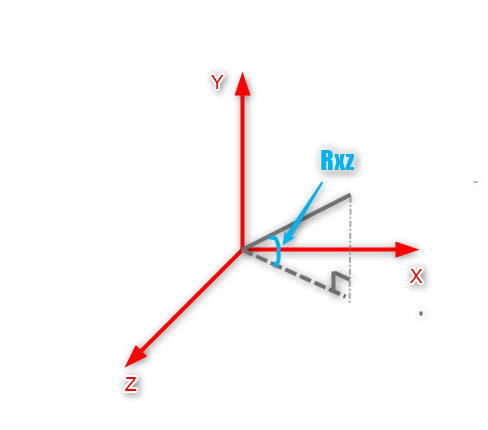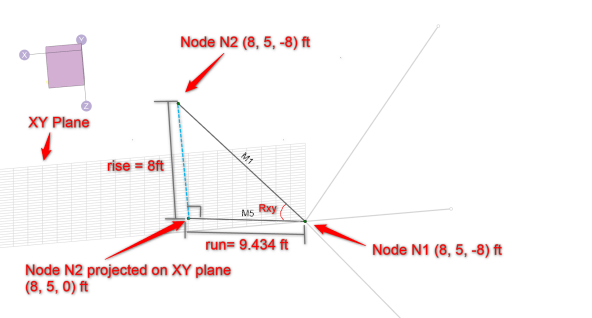When using the Distance tool in RISA-3D, the program outputs Rxz, Rzy, Ryz. These angles represent the angle the Distance Tool line makes with respect to the each respective plane. Rxy represents the angle the distance line makes with respect to the XY plane.

The Rxy, Rxz and Ryz angles refer to the angles for a member with respect to each 2-D plane defined by xyz. For instance, Angle Rxz refers to the angle between the member and the xz plane, i.e., angle between the member and its projection to xz plane.In the example below, we are interested in determining the Rxy angle. If we apply the software's theory to member M1, and project the member onto the XY plane, we can determine the angle the member makes with respect to the XY plane: